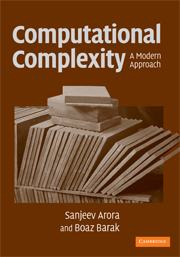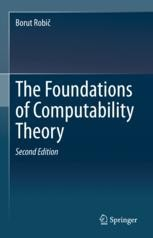Subscribe via Email

July 12, 2021 | 7 minutes to read

Resources for Learning Computational Complexity Theory

Computational complexity theory studies the feasibility of solving and resources required to solve computational problems and is useful to any field that thinks about the analysis and design of algorithms (which is much more broad than one may first think). While there are a good bit of notes and lectures available online, these are scattered across university course pages, YouTube, etc. This guide aims to bring this material together for learning computational complexity theory at the introductory graduate level, especially for those without a formal CS background.

Background Material

Computational complexity is a topic that goes as mathematically deep as one wants to go. At the edge of the shallow end, it’s helpful to be familiar with basic proof writing techniques and discrete math concepts. This is usually the prerequisite for a first undergraduate course in complexity theory and is often covered in appendices or chapter zero of introductory texts. Going a bit deeper to the graduate level, one will want some exposure to rigorous discrete math, particularly graphs and combinatorics with probability, as well as computability and models of computation.

For an introduction to discrete math, check out the relevant chapters from these lecture notes by James Aspnes. For a more indepth treatment of graph theory, see Reinhard Diestel’s Graph Theory text.

Harry Porter has an excellent set of video lectures on theoretical computer science. The first half begins with finite state machines and works up to Turing machines providing a thorough introduction to models of computation. The second half introduces computability theory as well as useful concepts used throughout theoretical computer science such as reduction.

BooksThe question of which books to read has received a lot of attention online. I recommend Arora and Barak’s Computational Complexity: A Modern Approach and Part 3 of Sipser’s Introduction to the Theory of Computation. Arora and Barak seems to be the consensus recommendation and offers clear, concise presentations of time and space complexity, hierarchy theorems, and circuit complexity as well as coverage of a wide variety of additional topics. Even better, there’s a pdf draft of Arora and Barak available on the book’s website.While Part 3 of Sipser covers fewer topics (it is just one part of a more comprehensive text), it contains more exposition on early topics than Arora and Barak. For instance, the Sipser text prefaces many proofs with the “proof idea” which provides helpful commentary on the proof strategy and techniques used. These are the sort of helpful comments that would be provided during a lecture but are often missing from the experience of working through a text independently. This makes Sipser a good secondary text even though it only covers the core material.

An alternative secondary text is Goldreich’s Computational Complexity: a Conceptual Perspective. Much like Sipser, Goldreich provides interesting and helpful exposition in places where Arora and Barak fall a bit short. On the book’s website, you can find a pdf draft (with only a few formatting issues).

Video Lectures

For some people (myself included), hearing and seeing someone walk through an example can be the difference between internalizing the example to build intuition and second-guessing the result each time a similar case comes up. The best comprehensive set of video lectures I’ve found is a mix from Ryan O’Donnell’s undergraduate and graduate computational complexity courses at CMU. As a rough guide, consider looking at the second half of the undergraduate course and the first half of the graduate course.

What’s Next?!

Getting a handle on the basics of rigorous computational complexity theory allows one to pursue numerous other areas of computer science, mathematics, and beyond. Below are some that I find interesting and recommendations for a first dive into each area.

Computational Learning TheoryComputational Learning Theory studies machine learning (usually supervised learning) using models and tools from theoretical computer science. Given a well-defined task and framework, this theory seeks to prove general statements along the lines of such-and-such a model class (e.g. linear classifiers) can solve the given task computationally feasibly (usually meaning polynomial time) with reasonable error. A good place to start is with the first half of Understanding Machine Learning. The second half (which is also worth reading) covers applications and advanced topics.

Cryptography

Cryptography is the mathematical study of secure communication. While there are various notions of security in cryptographic models, security is often defined as the absence of an adversary with such-and-such resources (usually restricted to polynomial time) that can learn such-and-such information.

A good reference here is Boneh and Shoup’s A Graduate Course in Applied Cryptography. Do note that this book can be quite terse and difficult. Consider The Joy of Cryptography for a more gentle introduction. Both books are available in pdf form on their respective websites.

Computability TheoryWhereas complexity theory categorizes computational problems by the resources required to solve them, one way to view computability theory (or recursion theory) is as categorizing computational problems by their degree of unsolvability.

There are several classic texts on computability theory such as Cutland’s Computability; however, the first book that I enjoyed working through was The Foundations of Computability Theory by Borut Robič. This book provides a gentle introduction to computability along with a good deal of historical context and an introduction to the philosophy of mathematics.

Descriptive Complexity

Descriptive Complexity looks at correspondences between complexity classes and definability in logics over finite structures. These results provide an alternative perspective on the relative difficulty of complexity classes as the expressivity required to define particular subsets of a finite structure. In this way, descriptive complexity is analogous to descriptive set theory or effective descriptive set theory. The typical reference for descriptive complexity is Neil Immerman’s Descriptive Complexity.

Mathematical Philosophy

Mathematical philosophy (also called formal philosophy or exact philosophy) considers philosophical questions by applying concepts and tools from mathematics and logic. The most popular area of formal philosophy at the moment is formal epistemology which - among other things - studies probabilistic and logical models of belief and decision.

In a wide-ranging survey article, Scott Aaronson makes the case that philosophy - and mathematical philosophy in particular - would benefit from considering matters of computational complexity in addition to computability. Aaronson speculates that philosophers grapple with computability rather than complexity since the former shares intellectual roots with interwar analytic philosophy and workings in the foundations of mathematics while the latter was developed later by computer scientists after the two diverged in interests.